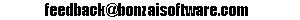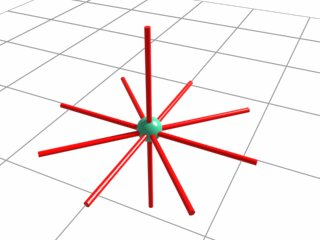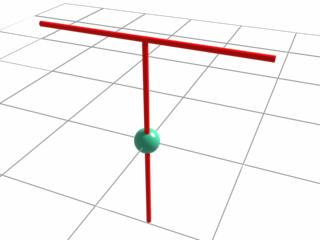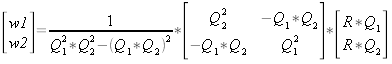# Collision Detection Tutorial

26.7.06

### Introduction

Welcome to my second tutorial! This time I’ll show you how the collision detection and response was performed in my demos. I don’t claim that it is the best method, but it does it’s job well and it should be easy to implement it. Since I’m not a math expert, I won’t explain all details of the used equations, but I’ll try to give you a basic understanding of what’s happening. However, I assume that you already understand how vectors and matrices work. As with everything on my site, please send your questions, critics or bugs toor visit the forum.

### Idea

The idea behind the algorithm is to place some lines “through” the camera like in figure 1. The more lines you have, the more precise is the result. The lines can be placed in any way you want, so you can perform the collision detection with all kinds of shapes (figure 2).Figure 1: line setup used for this tutorialFigure 2: line setup for a “T”-Shape
Then you check for each triangle in your scene if one of the lines collides with them. If it does, the camera is moved back. The lines will be represented by rays,so the problem simplifies to some ray-triangle intersection tests.

### Helper Functions

Here are some used functions which will make the code easier to read.

signum(): returns 1 if the parameter is negative, otherwise 0.
dot(): returns the dot product.
cross(): returns the cross product.
Normalize(): normalize vector.

```bool signum(float value)
{
if( value < 0.0f ) return 1;
else 		   return 0;
}

float dot( Vector3 v1, Vector3 v2)
{
return v1.x*v2.x + v1.y*v2.y + v1.z*v2.z;
}

Vector3 cross( Vector3 v1, Vector3 v2)
{
Vector3 ret;
ret.x =  v1.y*v2.z - v1.z*v2.y;
ret.y =  v1.z*v2.x - v1.x*v2.z;
ret.z =  v1.x*v2.y - v1.y*v2.x;
return ret;
}

void Vector3::Normalize()
{
float rcplen = 1.0f / sqrtf(x*x + y*y + z*z);
x*=rcplen:
y*=rcplen:
z*=rcplen:
}```

### Implementation

First you need to declare some variables which will be used later. cdA[] stores the lines which are placed “through” the camera. Since the used line setup is symetric, you only need to define starting points. You can later put a “-” before them to mirror them.
The parameter &CameraPosition is a reference to your camera position:

```void DoCollisionDetection( Vector3 &CameraPosition )
{
Vector3 vS, vV, vTV;
float t;
float s1, s2, mscale;
Vector3 vR, vQ1, vQ2;
Vector3 vIntersect;
Vector3 cV;

//starting points for
//line setup as shown in figure 1
Vector3 cdA;
cdA = Vector3( 0.5f, 0.0f, 0.0f );
cdA = Vector3( 0.0f, 0.5f, 0.0f );
cdA = Vector3( 0.0f, 0.0f, 0.5f );
cdA = Vector3( 0.4f, 0.0f, 0.4f );
cdA = Vector3(-0.4f, 0.0f, 0.4f );```

Then make a loop through all of your triangles and calculate the plane of each triangle. A plane is defined by a normal and the distance to the origin. A, B and C are often used as names for the x-, y- and z-components of the normal and D as a name for the distance to the origin. If you also call them like this, the plane equation is:

A*P.x + B*P.y + C*P.z + D = 0
which means:
dot(PlaneNormal, Point) + Distance_To_Origin = Distance_Point_To_Plane

Where P is a point on the plane. If the point P is exactly on the plane, the result of the equation (Distance_Point_To_Plane) is zero. If the Point is in front of the plane, the result is positive. If the Point is behind the plane, the result is negative.
For a better performance you should precalculate the plane equations for the static geometry during initialization. Note that the normal of the triangle is the same as the normal of the plane.

``` 	//loop through all triangles
for(int i=0; i < nNumberOfTriangles ; i++)
{
//normal of triangle and plane
Vector3 normal;

//points of triangle
Vector3 pot0 , pot1 , pot2;

pot0 = YourTriangles[i].Vertex;
pot1 = YourTriangles[i].Vertex;
pot2 = YourTriangles[i].Vertex;

//calculate normal
normal = cross(pot1-pot0, pot2-pot0);
//normalize normal
normal.Normalize();

//calculate distance to origin
float dist = -dot(normal, pot0);```

Now you can convert the lines to rays. Rays are defined by a starting point, a direction and an interpolation factor t in this equation:

PointOnRay = RayStartingPoint + RayDirection * t

Then you check if the starting point of each line is on the same side of the triangle plane as it’s end point. If both points are on the same side the line also can’t intersect the triangle and you don’t need to calculate anything. From the last step you know that the plane equation can be used to tell you if a point is on/behind/in front of the plane. This comes in handy now because you only need to plug in the points of the lines:

```for(int k=0; k<5; k++)
{

//line starting point and ray starting point
vS = CameraPosition + cdA[k];

//line end point
vV = CameraPosition - cdA[k];

//ray direction
vTV = vV - vS;

//Distance_Point_To_Plane =
//dot(PlaneNormal, Point)+Distance_To_Origin

//distance of starting point to plane
s1 = dot(normal, vS) + dist;
//distance of end point to plane
s2 = dot(normal, vV) + dist;

//the sign of s1 and s2 will tell
//you on which side the points are.
if( signum(s1) != signum(s2) )
{
//line intersects the plane of the triangle```

At this point you already know that the line intersects the plane of the triangle, but you still don’t know at which point. So you need to find the factor t of the ray equation for the intersection point:

```
//find the right factor
t = -s1 / dot(normal, vTV);

//calculate intersection point
vIntersect = vS + (vTV*t);```

The next step is to calculate if the intersection point vIntersect lies within the triangle. You can do this by using barycentric coordinates. With barycentric coordinates, every point on the triangle can be represented as a weighted average of the points (=the vertices) of the triangle:

PointOnTriangle = weight0 * Point0 + weight1 * Point1 + weight2 * Point2

Only if all weights aren’t negative, the point is inside the triangle, also the sum of all weights is exactly 1. This means you can calculate one of the weights by subtracting the other two from 1: weight0 = 1.0 – weight1 – weight2.
To find the two other weights, you have to solve this equation:Where
R = vIntersect – pot0 = weight1*Q1 + weight2*Q2;
Q1 = pot1-pot0;
Q2 = pot2-pot0;
Here is the code:

``````//calculations are performed relative to pot0
vR  = vIntersect - pot0;
vQ1 = pot1 - pot0;
vQ2 = pot2 - pot0;

float detp1 = dot(vQ1, vQ1)*dot(vQ2, vQ2);
float detp2 = dot(vQ1,vQ2)*dot(vQ1,vQ2);

mscale = 1/( detp1 - detp2);
float matrixA = { dot(vQ2, vQ2), -dot(vQ1, vQ2),
-dot(vQ1, vQ2),dot(vQ1, vQ1) };
float matrixB = {dot(vR, vQ1), dot(vR, vQ2)};

//invert first matrix
for(int j=0; j<4;j++) matrixA[j] *= mscale;

//multiply both matrices to get the weights
float w1= matrixA*matrixB + matrixA*matrixB;
float w2= matrixA*matrixB + matrixA*matrixB;

//calculate weight0
float w0 = 1.0f - w1 -w2;
``````

You now have everything you need to perform the collision detection and response. First you check if all weights are nonnegative to see if an intersection occured. If you found an intersection you calculate the offset to move the camera back. Since only 1 ray was used for each line, you also need to check if the intersection point is in the mirrored half. If it is, you need to get the offset between the line end point and the intersection point, otherwise you need the offset between the line starting point and the intersection point :

```//if all weights are nonnegative,
//the line intersects the triangle
if(signum(w0) == 0 && signum(w1) == 0 && signum(w2) == 0)
{
Vector3 offset;

//check which offset is needed
if(t>=0.5f) offset = vIntersect - vV;
else 	    offset = vIntersect - vS;

CameraPosition = CameraPosition + offset;```

Close the brackets:

```				}
}
}
}
}```

Done!

### Conclusion

The presented algorithm is suited for arbitrary objects in small scenes. There is still some space for optimizations to further reduce calculations, especially if you use some kind of spatial partitioning algorithm.
Discuss this tutorial in the forum